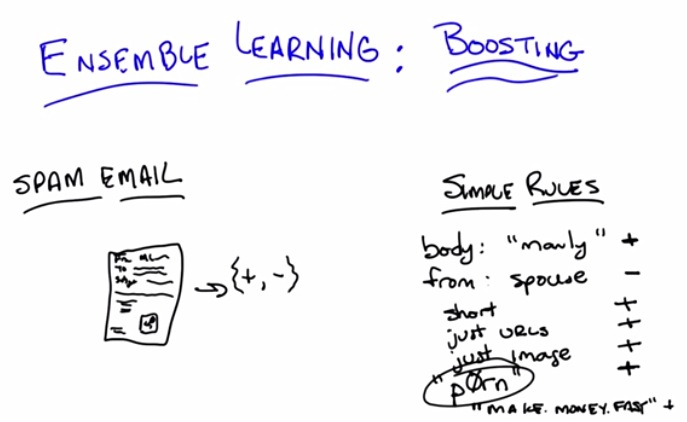• Now we're implementing something called spam classfier.
• Boosting is one of the famous ensemble learning
• First let's introduce a problem of spam email
• we can think of being positive as one indication of spam
• and negative as non spam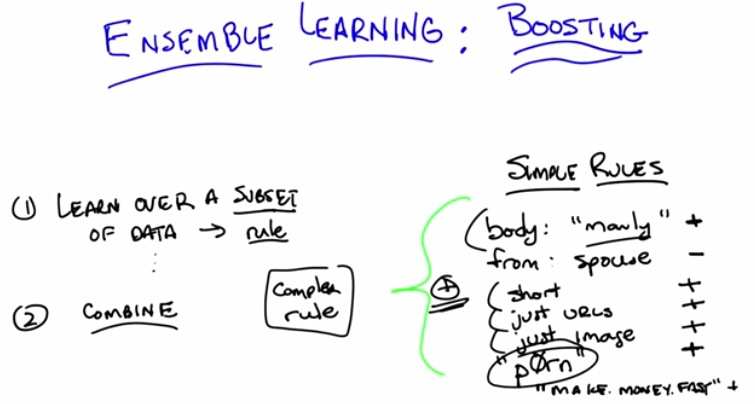• This is the core algorithm of boosting algorithm
• First learn a simple spam indicator. set that as a rule
• Then for every other spam, combine more and more until whole one rule
• But it's not be the case to include directly whole data as a rule. That would be called overfiting.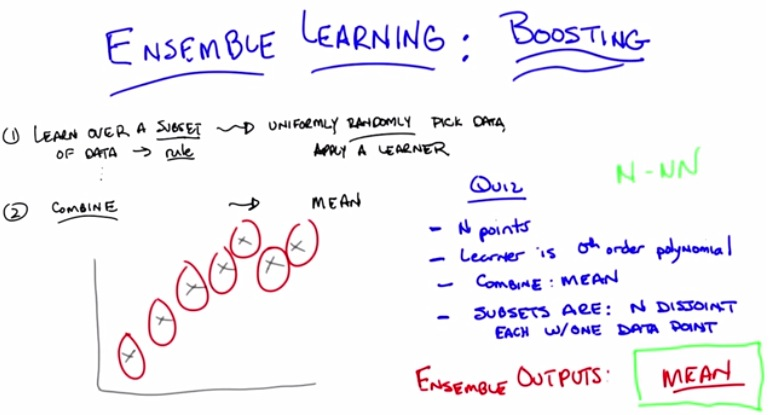• This is just how overall boosting should look like.
• One of the simplest method of 1 is just taking subset(email) and learn from it.
• Then for combine, just average of it
• So if we take it to the learning algorithm
• We have n points, and the attribute is all 0th order poloynomial.
• We take one data point and take to the learner, the results is the mean for one particuular data points.
• The ensemble outputs are just mean with n-numbers.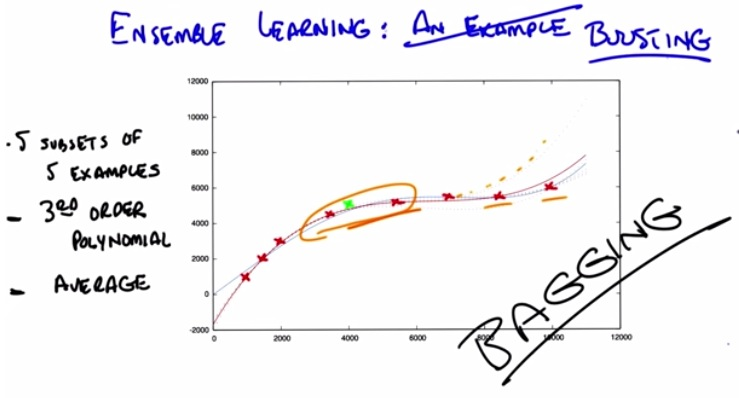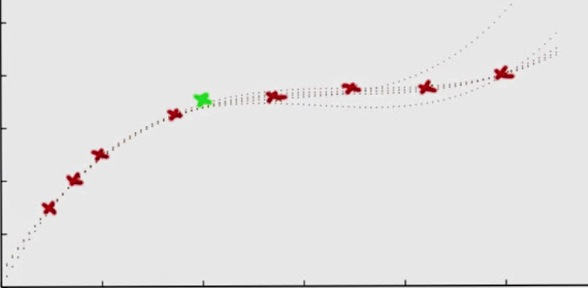• First we take randomly 5 subsets; each subset takes randomly 5 example from it.
• the red points is the all available data points, and the green one is cross validation points
• Then we apply third order polynomial, and average each of the subsets.
• The curve will then produced 5 lines.
• We can see that all line perform polynomial regression.
• Some of the lines match point 1 to 4, but last two points maybe missed
• The red line is the average of all subsets with 3rd degree polynomial
• The blue line is the average of all subsets with 4th degree polynomial
• These overall called bagging, takes bag of data, but not necessarily called boosting.